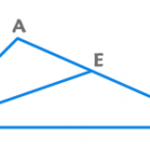Get a better understanding of triangle related questions in CAT | SDA Bocconi Asia Center
Call Us Now+91-2240867000    Back to Main Website

# Get a better understanding of triangle related questions in CAT

Geometry is probably the most important topic when it comes to CAT preparation. For some reason, a large amount of time during CAT preparation, online as well as offline, is spent discussing topics like Remainders and Probability which are not that important from CAT perspective. On the other hand, triangles are a very common figure if you consider the CAT exam. You will find questions based on a variety of polygons being solved on the basis of properties of triangles. In this particular post, I will try to cover some of the most relevant tips and tricks when it comes to triangles.

Basic Properties of a Triangle

Some of the obvious basic properties of a triangle are:

1. The Sum of interior angles of a triangle is 180° and sum of exterior angles is 360°.
2. Exterior Angle = Sum of remote interior angles.
3. The Sum of two sides is always greater than the third side and the difference of two sides is always lesser than the third side.
4. The Side opposite to the biggest angle is longest and the side opposite to the smallest angle is the shortest.

Quite often, a few options can be eliminated just be applying these properties.

Area of a Triangle= ½ x Base x Height

= ½ x Product of sides x Sine of included angleSpecial Points in a triangle

While I am sure you would know what the Centroid / Incenter / Circumcenter / Orthocenter are, here are some special properties related to them that can help you lead to the answers quickly.

• Centroid and Incenter will always lie inside the triangle.
• For an acute angled triangle, the Circumcenter and the Orthocenter will lie inside the triangle.
• For an obtuse angled triangle, the Circumcenter and the Orthocenter will lie outside the triangle.
• For a right angled triangle, the Circumcenter will lie at the midpoint of the hypotenuse and the Orthocenter will lie at the vertex at which the angle is 90°.

Theorems related to triangles

While there are multiple theorems that are related to the triangles, there are some that are less popular. If you incorporate these while solving questions, you will be able to reach the answer quickly.Apollonius’ Theorem: AB2 + AC2 = 2 (AD2 + BD2)Interior Angle Bisector Theorem: AE/ED = BA/BD

Here BE is the angle bisector

Integer Triangles: The number of integer triangles is the integer closest to p^2/48 when p is even and to (p+3)^2/48 when p is odd

I hope you would have learned something from this post about Triangles which forms an integral part of CAT Preparation. Do provide feedback about the same via the comments section on the blog. I look forward to your suggestions.

Ravi Handa, an alumnus of IIT Kharagpur, has been teaching for CAT and various other competitive exams for around a decade. He started online courses on his website Handa Ka Funda in 2013 and 10000+ students have subscribed for them since then.

Tags: , ,

1 Comment

•kirti pandey

Sir you r too good , your suggestions helped me very much during the entire preparation.